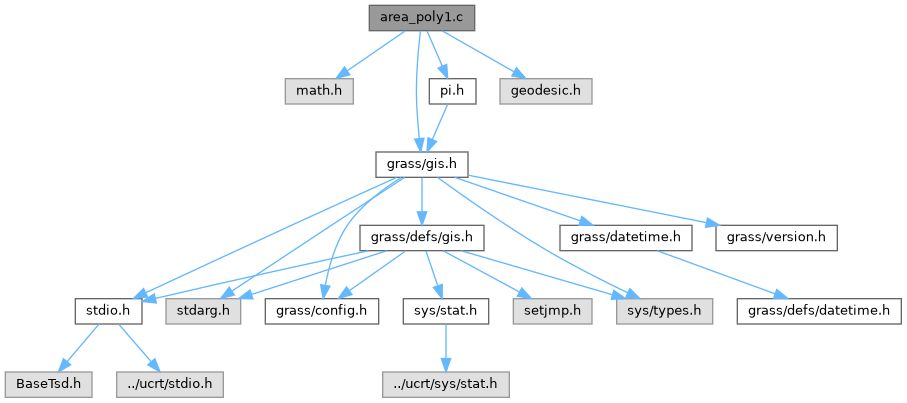GRASS GIS 8 Programmer's Manual  8.2.2dev(2023)-5c00b4e944
area_poly1.c File Reference

GIS Library - Polygon area calculation routines. More...

`#include <math.h>`
`#include <grass/gis.h>`
`#include "pi.h"`
Include dependency graph for area_poly1.c:Go to the source code of this file.

## Macros

#define TWOPI   M_PI + M_PI

## Functions

void G_begin_ellipsoid_polygon_area (double a, double e2)
Begin area calculations. More...

double G_ellipsoid_polygon_area (const double *lon, const double *lat, int n)
Area of lat-long polygon. More...

## Detailed Description

GIS Library - Polygon area calculation routines.

(C) 2001-2013 by the GRASS Development Team

This program is free software under the GNU General Public License (>=v2). Read the file COPYING that comes with GRASS for details.

Definition in file area_poly1.c.

## ◆ TWOPI

 #define TWOPI   M_PI + M_PI

Definition at line 18 of file area_poly1.c.

## ◆ G_begin_ellipsoid_polygon_area()

 void G_begin_ellipsoid_polygon_area ( double a, double e2 )

Begin area calculations.

This initializes the polygon area calculations for the ellipsoid with semi-major axis a (in meters) and ellipsoid eccentricity squared e2.

Parameters
 a semi-major axis e2 ellipsoid eccentricity squared

Definition at line 61 of file area_poly1.c.

## ◆ G_ellipsoid_polygon_area()

 double G_ellipsoid_polygon_area ( const double * lon, const double * lat, int n )

Area of lat-long polygon.

Returns the area in square meters of the polygon described by the n pairs of lat,long vertices for latitude-longitude grids.

Note: This routine computes the area of a polygon on the ellipsoid. The sides of the polygon are rhumb lines and, in general, not geodesics. Each side is actually defined by a linear relationship between latitude and longitude, i.e., on a rectangular/equidistant cylindrical/Plate Carr{'e}e grid, the side would appear as a straight line. For two consecutive vertices of the polygon, (lat_1, long1) and (lat_2,long_2), the line joining them (i.e., the polygon's side) is defined by:

```                                    lat_2  -  lat_1
lat = lat_1 + (long - long_1) * ---------------
long_2 - long_1
```

where long_1 < long < long_2. The values of QbarA, etc., are determined by the integration of the Q function. Into www.integral-calculator.com, paste this expression :

```sin(x)+ (2/3)e^2(sin(x))^3 + (3/5)e^4(sin(x))^5 + (4/7)e^6(sin(x))^7
```

and you'll get their values. (Last checked 30 Oct 2013).

This function correctly computes (within the limits of the series approximation) the area of a quadrilateral on the ellipsoid when two of its sides run along meridians and the other two sides run along parallels of latitude.

Parameters
 lon array of longitudes lat array of latitudes n number of lat,lon pairs
Returns
area in square meters

Definition at line 129 of file area_poly1.c.

Referenced by G_area_of_polygon().